Practice Test: Mechanical Engineering (ME)- 7

# Practice Test: Mechanical Engineering (ME)- 7 - Mechanical Engineering

Test Description

## 65 Questions MCQ Test GATE Mechanical (ME) 2024 Mock Test Series - Practice Test: Mechanical Engineering (ME)- 7

Practice Test: Mechanical Engineering (ME)- 7 for Mechanical Engineering 2023 is part of GATE Mechanical (ME) 2024 Mock Test Series preparation. The Practice Test: Mechanical Engineering (ME)- 7 questions and answers have been prepared according to the Mechanical Engineering exam syllabus.The Practice Test: Mechanical Engineering (ME)- 7 MCQs are made for Mechanical Engineering 2023 Exam. Find important definitions, questions, notes, meanings, examples, exercises, MCQs and online tests for Practice Test: Mechanical Engineering (ME)- 7 below.
Solutions of Practice Test: Mechanical Engineering (ME)- 7 questions in English are available as part of our GATE Mechanical (ME) 2024 Mock Test Series for Mechanical Engineering & Practice Test: Mechanical Engineering (ME)- 7 solutions in Hindi for GATE Mechanical (ME) 2024 Mock Test Series course. Download more important topics, notes, lectures and mock test series for Mechanical Engineering Exam by signing up for free. Attempt Practice Test: Mechanical Engineering (ME)- 7 | 65 questions in 180 minutes | Mock test for Mechanical Engineering preparation | Free important questions MCQ to study GATE Mechanical (ME) 2024 Mock Test Series for Mechanical Engineering Exam | Download free PDF with solutions
 1 Crore+ students have signed up on EduRev. Have you?
Practice Test: Mechanical Engineering (ME)- 7 - Question 1

### Choose the most approximate word from the options given below to complete the following sentence. If I had known that you were coming, I _______ you at the airport

Detailed Solution for Practice Test: Mechanical Engineering (ME)- 7 - Question 1

The sentence talks about a past incident and a condition has been mentioned. Thus option 2 fits here correctly. 'Would have' is the correct tense to be used here. Option 1 is illogical. 'Would' must be used here and not 'will' as it is in the past tense. Past perfect tense is incorrect here.

Practice Test: Mechanical Engineering (ME)- 7 - Question 2

### Choose the most approximate word from the options given below to complete the following sentence. I believe in the _____ of positive thinking thus always recommend these books to my clients.

Detailed Solution for Practice Test: Mechanical Engineering (ME)- 7 - Question 2

The word that fits here is a noun thus options 3 and 4 are eliminated. The form should be singular as for abstract nouns we do not use the plural form. Option 1 is thus the correct answer.

The word powerful is an adjective and 'empowering' is a verb.

Practice Test: Mechanical Engineering (ME)- 7 - Question 3

### Consider a circle of radius r. Fit the largest possible square inside it and the largest possible circle inside the square. What is the radius of the innermost circle?

Detailed Solution for Practice Test: Mechanical Engineering (ME)- 7 - Question 3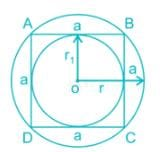The radius of outer circle = r

∴ Diagonal of square = 2r

Side of square (a)=√2r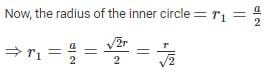Practice Test: Mechanical Engineering (ME)- 7 - Question 4

A bird files along the three sides of a field in the shape of an equilateral triangle at speeds of 3, 6, 8 km/hr respectively. The average speed of the bird is

Detailed Solution for Practice Test: Mechanical Engineering (ME)- 7 - Question 4

Average speed = Total distance/Total time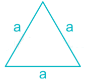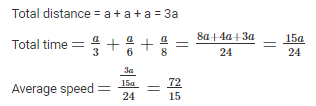Practice Test: Mechanical Engineering (ME)- 7 - Question 5

Choose the most appropriate words from the options given below to fill in the blanks.

She was ______ to travel abroad and _____ in the field of commerce as per her wishes.

Detailed Solution for Practice Test: Mechanical Engineering (ME)- 7 - Question 5

The sentence mentions a person doing something as per her wishes thus the first word must be a positive reaction. 'Restive' which means 'restless' and 'jinxed' which means 'cursed' are incorrect here. Between options 1 and 3,the word 'elated' which means 'too happy' and the word 'venture' which means 'undertake a risky or daring journey or course of action' fit here correctly. The word 'cease' means 'stop' and does not convey a proper meaning. Thus option 3 is the correct answer.

Practice Test: Mechanical Engineering (ME)- 7 - Question 6

In a 1600 m race around a circular track of length 400 m, the faster runner and the slowest runner meet at the end of the sixth minute, for the first time after the start of the race. All the runners maintain uniform speed throughout the race. If the faster runner runs at twice the speed of the slowest runner. Find the time taken by the faster runner to finish the race

Detailed Solution for Practice Test: Mechanical Engineering (ME)- 7 - Question 6

As, the faster runner is twice as fast as the slowest runner, the faster runner would have complete two rounds by the time the slowest runner completes one round.

Their first meeting takes place after the fastest runner takes 6 min to complete two rounds and the slowest runner completes one round.

Time taken to complete one round by fastest runner = 6/2 = 3 minutes

Rounds needed to complete the race = 1600/400 = 4

Time taken to complete the race = 4 × 3 = 12 min

Practice Test: Mechanical Engineering (ME)- 7 - Question 7

Select the pair that best expresses a relationship similar to that expressed in the pair:

Horse: Foal

Detailed Solution for Practice Test: Mechanical Engineering (ME)- 7 - Question 7

The young one of a horse is called a foal. Similarly, the young one of a goose is known as gosling. Thus, option 2 contains the pair that best expresses a relationship similar to that expressed in the given pair. In all the other pairs, the first word expresses the male form of the latter.

Practice Test: Mechanical Engineering (ME)- 7 - Question 8

Juvenile delinquency is also termed as Teenage Crime. Basically, juvenile delinquency refers to the crimes committed by minors. These crimes are committed by teenagers without any prior knowledge of how it affects the society. These kind of crimes are committed when children do not know much about outside world.

Which of the following Inferences is correct with respect to above passage?

Detailed Solution for Practice Test: Mechanical Engineering (ME)- 7 - Question 8

Since teenagers here have tender age and commit crimes unknown to its consequences, clearly they should not be treated as any criminal. They need to be taught so that they can make difference between right and wrong and do not commit these crimes in future. Hence inference in option 1 is correct.

Practice Test: Mechanical Engineering (ME)- 7 - Question 9

If n and y are positive integers and 450 y = n³, which of the following must be an integer?

Detailed Solution for Practice Test: Mechanical Engineering (ME)- 7 - Question 9

450 y = n³ implies that 450 y is the cube of an integer.

When we prime-factorize the cube of an integer, we get 3 (or a multiple of 3) of every prime factor.

8 is the cube of an integer because 8 = 2³ = 2*2*2.

Thus, when we prime-factorize 450 y, we need to get at least 3 of every prime factor.

Here's the prime factorization of 450 y:

450 y = 2 * 3² * 5² * y

Since 450 provides only one 2, two 3's, and two 5's, and we need at least 3 of every prime factor, the missing prime factors must be provided by y. Thus, y must provide at at least two more 2's, one more 3, and one more 5.

Smallest possible case:

y = 2² * 3 * 5

Practice Test: Mechanical Engineering (ME)- 7 - Question 10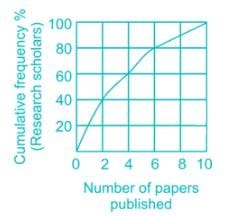The graph shows cumulative frequency % of research scholars and the number of papers published by them. Which of the following statements is true?

Practice Test: Mechanical Engineering (ME)- 7 - Question 11

For which value of x, the following matrix A is a singular matrix.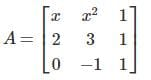Practice Test: Mechanical Engineering (ME)- 7 - Question 12

Find the laplace transform of t2sin (2t).

Practice Test: Mechanical Engineering (ME)- 7 - Question 13

Consider the following function: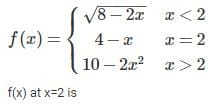Practice Test: Mechanical Engineering (ME)- 7 - Question 14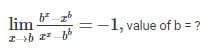Practice Test: Mechanical Engineering (ME)- 7 - Question 15

The integrating factor of equation y log y dx + (x – log y) dy = 0 is

Practice Test: Mechanical Engineering (ME)- 7 - Question 16

The Mohr’s circle given below corresponds to which one of the following stress condition.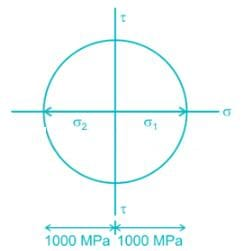Practice Test: Mechanical Engineering (ME)- 7 - Question 17

For a laminar flow over a flat plate, the local heat transfer coefficient ‘hx’ varies as x-1/2, where x is the distance from the leading edge (x = 0) of the plate. The ratio of the average coefficient ‘ha’ between the leading edge and some location ‘A’ at x = x on the plate to the local heat transfer coefficient ‘hx’ at A is:

Practice Test: Mechanical Engineering (ME)- 7 - Question 18

A vapour absorption refrigeration system is a heat pump with three thermal reservoirs as shown in the figure. A refrigeration effect of 100 W is required at 250 K when the heat source available is at 400 K. Heat rejection occurs at 300 K. The minimum value of heat required (in W) is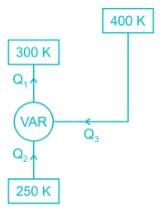*Answer can only contain numeric values
Practice Test: Mechanical Engineering (ME)- 7 - Question 19

The heat loss from a fin is 8 W. The effectiveness and efficiency of the fin are 4 and 0.8, respectively. The heat loss (in W) from the fin, keeping the entire fin surface at base temperature, is ________.

Practice Test: Mechanical Engineering (ME)- 7 - Question 20

An activity has an early start date of the 10th and a late start date of the 19th. The activity has a duration of four days. There are no non-workdays. From the information given, what can be concluded about the activity?

Practice Test: Mechanical Engineering (ME)- 7 - Question 21

In Fig. 1-12, assume that a 20-mm-diameter rivet joins the plates that are each 110 mm wide. The allowable stresses are 120 MPa for bearing in the plate material and 60 MPa for shearing of rivet. Determine (a) the minimum thickness of each plate; and (b) the largest average tensile stress in the plates.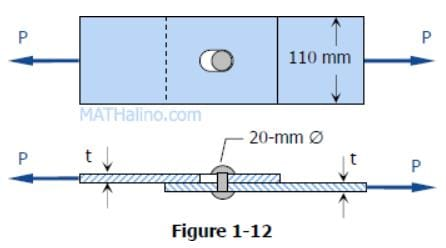Practice Test: Mechanical Engineering (ME)- 7 - Question 22

The coeffcient of thermal expansion of steel is 12 × 10-6/°C and that of stainless steel is 18 × 10-6/°C. Length of steel bar is twice the length of stainless steel bar. The ratio of expansion of steel bar to expansion of stainless steel bar, when there is rise of temperature is-

Practice Test: Mechanical Engineering (ME)- 7 - Question 23

At room temperature, pure titanium possesses which of the following crystal structure _______?

*Answer can only contain numeric values
Practice Test: Mechanical Engineering (ME)- 7 - Question 24

A 400 mm long sprue carries molten metal to fill a cylindrical mould of diameter 20 mm & height of 30 mm by top gating system. If the molten metal flow rate through the sprue is 600 mm3/sec, & it is maintained constant by maintaining constant metal head in pouring basin. Then find the mould filling time (in Seconds) ________?

Practice Test: Mechanical Engineering (ME)- 7 - Question 25

A cup is drawn in a deep drawing operation. The inside diameter of cup is 100 mm. If sheet thickness and blank diameter is 4 mm and 240 mm respectively then find percentage reduction ________?

*Answer can only contain numeric values
Practice Test: Mechanical Engineering (ME)- 7 - Question 26

A turbine develops 900 kW when running at 200 r.p.m. The head on the turbine is 30 m. If the head on the turbine is reduced to 18 m, determine the speed of the turbine (in rpm).

*Answer can only contain numeric values
Practice Test: Mechanical Engineering (ME)- 7 - Question 27

The frequency of a spring-mass suspension becomes 60 percent of the resonant frequency when a damper is added to it. What will be the damping ratio?

Practice Test: Mechanical Engineering (ME)- 7 - Question 28

Square blanks of 8 mm side lengths are blanked from an aluminium sheet of 3 mm thickness. The shear strength of the aluminium is 85 MPa. The minimum blanking force (in kN) is _________?

Practice Test: Mechanical Engineering (ME)- 7 - Question 29

A cold rolled steel shaft is designed on the basis of maximum shear stress theory. The principal stresses induced at its critical section are 60 MPa and -60 MPa respectively. If the yield stress for the shaft material is 360 MPa, the factor of safety of the design is:

Practice Test: Mechanical Engineering (ME)- 7 - Question 30

Which of following related fluid flow parameters exist both in rotational and irrotational flows?

Practice Test: Mechanical Engineering (ME)- 7 - Question 31

The thickness of a plate is reduced from 30 mm to 10 mm by successive cold rolling passes using identical rolls of diameter 600 mm. Assume that there is no change in width. If the coefficient of friction between the rolls and work piece is 0.1. The minimum number of passes required is:

*Answer can only contain numeric values
Practice Test: Mechanical Engineering (ME)- 7 - Question 32

If the electro chemical machining (ECM) is to be used to cut pure iron with MRR of 800 mm3/min. Estimate the current required (in Ampere) _______, if given atomic mass of iron is 56 gm, ρFe = 7.8 gm/cc & valency is 2

Practice Test: Mechanical Engineering (ME)- 7 - Question 33

A rectangular block of dimensions 2 m × 1 m × 1 m is floating in the water with immersing depth of 0.5 m. What is the weight of block (kN) if unit weight of water is 10 kN/cubic meter.

Practice Test: Mechanical Engineering (ME)- 7 - Question 34

For the following hole and shaft sizes of mated parts according to basic hole system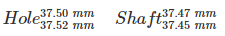What is the value of allowance?

*Answer can only contain numeric values
Practice Test: Mechanical Engineering (ME)- 7 - Question 35

In a counter flow heat exchanger, the effectiveness is given as 0.8. The hot and cold fluid are both air with equal mass flow rate. Find the number of transfer units of the heat exchanger.

Practice Test: Mechanical Engineering (ME)- 7 - Question 36

A block is displaced by 3 m when a force of 200 N is applied on it on an inclined surface which is at an angle of 50o with the horizontal. What is the work done?

Detailed Solution for Practice Test: Mechanical Engineering (ME)- 7 - Question 36

Given: Force = 200 N, θ = 50°, block displacement (D) = 3m
Formula: Work done = Force x cos θ x D
Work done = Force x cos θ x D
= 200 x cos 50 x 3 = 385.67 Nm
Work done = 385.67 Nm

*Answer can only contain numeric values
Practice Test: Mechanical Engineering (ME)- 7 - Question 37

The value of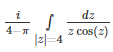is equal to ______.

*Answer can only contain numeric values
Practice Test: Mechanical Engineering (ME)- 7 - Question 38

Let y(x) be the solution of the initial value problem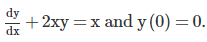Find the value of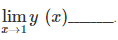(Correct up to 2 decimal places).

Practice Test: Mechanical Engineering (ME)- 7 - Question 39

2000 cashew nuts are mixed thoroughly in flour. The entire mixture is divided into 1000 equal parts. And each part is used to make 1 biscuit. Assume that no cashews are broken in the process. A biscuit is picked at random. The probability it contents no cashew is _____.

Practice Test: Mechanical Engineering (ME)- 7 - Question 40

If the following formula is used for the numerical integration of a function f(x), then what should be the values of the constants a, b, c so that for f(x) = 1 or f(x) =  x or f(x) =  x2, there is no error in the formula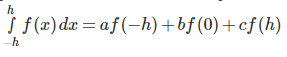Practice Test: Mechanical Engineering (ME)- 7 - Question 41

For a cantilever beam as shown in the figure, the segment PQ has flexural rigidity EI and segment QR has infinite flexural rigidity. The deflection and slope of the beam at Q are___________(respectively).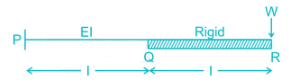*Answer can only contain numeric values
Practice Test: Mechanical Engineering (ME)- 7 - Question 42

The mass of each ball of a Proell Governor is 3 kg and the weight on the sleeve is 20 kg. Each arm is 220 mm long and the pivots of upper and lower arms are 20 mm from the axis. For the mid position of the sleeve, the extension links of the lower arms are vertical, the height of the governor is 180 mm, the speed is 150 rpm and the radius of path of rotation of the masses is 147 mm. Determine the lengths of the extension link (in mm).

Practice Test: Mechanical Engineering (ME)- 7 - Question 43

A Carnot engine operates between temperature 1000 K and 400 K. The heat rejected by the first Carnot engine is used by the second Carnot engine, whose sink temperature is 200 K. If the net heat absorbed by the first Carnot engine is 200 MJ. What is the heat rejected (in MJ) by the second Carnot engine?

Practice Test: Mechanical Engineering (ME)- 7 - Question 44

If two equal forces of magnitude P acts at an angle θ, their resultant will be

Practice Test: Mechanical Engineering (ME)- 7 - Question 45

In a reverted gear train the pinion (20 teeth) drives a gear B (60 teeth). The gear B and pinion C (15 teeth) are compounded. The pinion C drives the output gear D (45 teeth). The module for the first stage is 3. The module for the second stage and the velocity ratio are respectively:

Practice Test: Mechanical Engineering (ME)- 7 - Question 46

A heat engine receives heat from a source at 1500 K at a rate of 600 kW and rejects the waste heat to a medium at 350 K. If the power output from the heat engine is 250 kW, the irreversibility rate for the process is

*Answer can only contain numeric values
Practice Test: Mechanical Engineering (ME)- 7 - Question 47

A cylindrical shell 90 cm long and 20 cm internal diameter having thickness of metal as 8 mm is filled with fluid at atmospheric pressure. If an additional 20 cm3 of fluid is pumped into the cylinder, find the pressure (in MPa) exerted by the fluid on the cylinder. Take E = 2 × 105 N/mm2 and μ = 0.3.

Practice Test: Mechanical Engineering (ME)- 7 - Question 48

If 1.5 is the polytrophic index of compression for a reciprocating compressor which compresses air from 1 bar to 15.62 bar and the volume flow rate of air at inlet to the compressor is 5 m3/min then work input to the compressor (in kW) is

*Answer can only contain numeric values
Practice Test: Mechanical Engineering (ME)- 7 - Question 49

The compression ratio of air-standard Otto cycle is 9.5. Prior to the isentropic compression process, the air is 100 kPa, 35°C and 600 cm3. The temperature at the end of the isentropic expansion process is 800 K. Property data for air: Cp = 1.005 kJ/kg K, Cv = 0.718 kJ/kg K, R = 0.287 kJ/kg K. The highest pressure in the cycle is (in MPa) ________.

*Answer can only contain numeric values
Practice Test: Mechanical Engineering (ME)- 7 - Question 50

A crank rocker mechanism ABCD has dimensions AB = 30 mm, BC = 90 mm, CD = 75 mm and AD (fixed link) = 100 mm. Maximum value of transmission angle will be (in degree) ______.

*Answer can only contain numeric values
Practice Test: Mechanical Engineering (ME)- 7 - Question 51

The Dry Bulb temp and the wet bulb temp of moist air are 30°C and 20°C respectively. The atmospheric pressure is 740 mm of Hg. (Use g = 9.8 m/s2)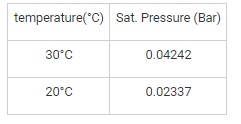*Answer can only contain numeric values
Practice Test: Mechanical Engineering (ME)- 7 - Question 52

Steam enters an engine at a pressure of 10 bar and 400°C. It is exhausted at 0.2 bar. The steam at exhaust is 0.9 dry. Find the change in entropy (in J/kg K).

Properties of steam and water is given as:

At 10 bar: Tsat = 179.91°C, v= 0.001127 m3/kg, vg = 0.19444 m3/kg, uf = 761.67 kJ/kg, ufg = 1822 kJ/kg, hf = 762.79 kJ/kg, hfg = 2015.3 kJ/kgm, sf = 2.1386 kJ/kg K, sfg = 6.5864 kJ/kg K

At 0.2 bar: hf = 251.5 kJ/kg, hfg = 2358.4 kJ/kg, sf = 0.8321 kJ/kg K, sg = 7.9094 kJ/kg K

At 10 bar and 400°C : h = 3263.9 kJ/kg, s = 7.465 kJ/kg K

*Answer can only contain numeric values
Practice Test: Mechanical Engineering (ME)- 7 - Question 53

A company has an annual demand of 2000 units, ordering cost is Rs. 100 per order & carrying cost is Rs. 8 per unit year, the shortage cost is Rs. 20 per unit year. The amount of shortage will be:

Practice Test: Mechanical Engineering (ME)- 7 - Question 54

The maximum work developed by a closed cycle used in a gas turbine plant when it is working between 900 K and 289 K and using air as working substance is:

Practice Test: Mechanical Engineering (ME)- 7 - Question 55

A piping system consists of three pipes arranged in series, the lengths of the pipes are 1200 m, 750 m and 600 m and diameters 750 mm, 600 mm, 450 mm respectively. Transform the system to an equivalent 450 mm diameter pipe

*Answer can only contain numeric values
Practice Test: Mechanical Engineering (ME)- 7 - Question 56

A circular disc mounted on a shaft carries three attached masses of 4kg, 3kg and 2.5 kg at radial distance of 75 mm, 85 mm and 50mm and at the angular positions of 45°, 135° and 240° respectively. The angular positions are measured counter clockwise from the reference line along the x - axis. The amount of the counter mass at a radial distance of 70mm required for the static balance will be ________ (in kg)

*Answer can only contain numeric values
Practice Test: Mechanical Engineering (ME)- 7 - Question 57

A U-tube shown in figure is filled with a liquid of relative density 1.25 to a height of 15 cm in both the limbs. It is rotated about a vertical axis 15 cm from limb and 30 cm from other. If the speed of rotation is 60 rpm, find the difference in liquid levels is 2 limbs (in cm).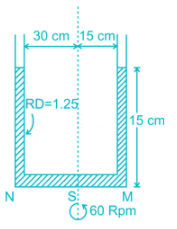*Answer can only contain numeric values
Practice Test: Mechanical Engineering (ME)- 7 - Question 58

A company has four jobs V, W, Z and Y and four machines A, B, C and D. The given matrix shows the return in Rs. of assigning a job to the machine. Assign the jobs to machines so as to maximize the total returns.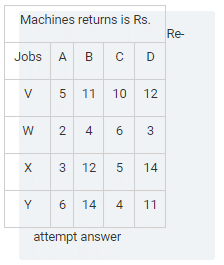*Answer can only contain numeric values
Practice Test: Mechanical Engineering (ME)- 7 - Question 59

Three marks A, B and C at a distance of 100 m each are made along a straight road. A car starting from rest and with uniform acceleration passes the mark A and takes 10 seconds to reach B and further 8 seconds to reach the mark C. What will be the velocity (in m/s) of car of A.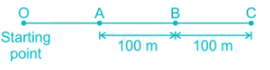*Answer can only contain numeric values
Practice Test: Mechanical Engineering (ME)- 7 - Question 60

Consider the rectangular tank filled with oil of specific gravity 1.83 up to certain height as shown in figure below.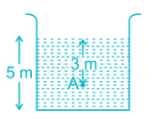It the atmospheric pressure is equal to 76cm of mercury. Then determine how much percentage (%) of absolute pressure is gauge pressure at point A. Take Relative density of mercury and water as 13.6 and 1 respectively.

*Answer can only contain numeric values
Practice Test: Mechanical Engineering (ME)- 7 - Question 61

A metal piece of length 60 cm has a cross section corresponding to a sector of a circle of radius 10 cm and included angle 60°. Its ends are maintained at 125°C and 25°C and thermal conductivity of the material has a linear variation with temp as (K = 100 - 0.01T) kcal/m - hr°C. The amount of heat flow (in kcal/hr) through the metallic piece is _______

(Neglect heat transfer in radial and angular direction)

*Answer can only contain numeric values
Practice Test: Mechanical Engineering (ME)- 7 - Question 62

A machine having mass of one tonne is mounted on the spring having stiffness of 2000 kN/m and damper having damping coefficient of 1050 N sec/m. The machine is subjected to external disturbing harmonic force of 0.8 kN at the frequency of 18.85 rad/s. The force transmitted to the foundation will be ________ (N)

Practice Test: Mechanical Engineering (ME)- 7 - Question 63

An electrician repairs geyser. He finds that the time he spends on repair of a geyser is exponentially distributed with mean 15 minutes. The geysers are required in the order in which these are received & their arrival approximate Poisson’s distribution with an average rate 24 per 8 hours day. Find electrician’s idle time each day (in minutes) _______?

*Answer can only contain numeric values
Practice Test: Mechanical Engineering (ME)- 7 - Question 64

A solid shaft of diameter 40 mm is used in power transmission. Due to modification of existing transmission system, it is required to replace the solid shaft by a hollow shaft of same material & equally strong in torsion. Find the outer diameter of hollow shaft (in mm)_______, if ratio of inner diameter to outer diameter is 0.6.

*Answer can only contain numeric values
Practice Test: Mechanical Engineering (ME)- 7 - Question 65

An orthogonal cutting operation is being carried out in which uncut chip thickness is 0.40 mm, cutting speed is 160 m/min, rake angle is 12°. It was observed that the chip thickness is 1.6 mm, the cutting force is 80 N & the thrust force is 20 N. Find the shear power ­­________ (in kW)?

## GATE Mechanical (ME) 2024 Mock Test Series

27 docs|243 tests
Information about Practice Test: Mechanical Engineering (ME)- 7 Page
In this test you can find the Exam questions for Practice Test: Mechanical Engineering (ME)- 7 solved & explained in the simplest way possible. Besides giving Questions and answers for Practice Test: Mechanical Engineering (ME)- 7, EduRev gives you an ample number of Online tests for practice

## GATE Mechanical (ME) 2024 Mock Test Series

27 docs|243 tests